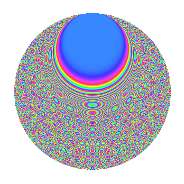# Properties

 Label 3009.2.nLevel 3009 Weight 2 Character orbit n Rep. character $$\chi_{3009}(178,\cdot)$$ Character field $$\Q(\zeta_{8})$$ Dimension 704 Sturm bound 720

# Related objects

## Defining parameters

 Level: $$N$$ = $$3009 = 3 \cdot 17 \cdot 59$$ Weight: $$k$$ = $$2$$ Character orbit: $$[\chi]$$ = 3009.n (of order $$8$$ and degree $$4$$) Character conductor: $$\operatorname{cond}(\chi)$$ = $$17$$ Character field: $$\Q(\zeta_{8})$$ Sturm bound: $$720$$

## Dimensions

The following table gives the dimensions of various subspaces of $$M_{2}(3009, [\chi])$$.

Total New Old
Modular forms 1456 704 752
Cusp forms 1424 704 720
Eisenstein series 32 0 32

## Trace form

 $$704q + 16q^{5} + 16q^{6} + O(q^{10})$$ $$704q + 16q^{5} + 16q^{6} - 16q^{11} - 752q^{16} + 32q^{19} - 32q^{20} + 48q^{22} - 16q^{24} + 32q^{25} + 32q^{26} + 16q^{28} - 16q^{29} + 16q^{31} - 80q^{32} + 16q^{33} - 16q^{34} - 16q^{36} + 32q^{37} - 32q^{40} + 16q^{41} - 48q^{42} - 16q^{43} + 16q^{45} + 32q^{46} + 16q^{49} - 64q^{52} + 48q^{53} + 16q^{54} + 112q^{56} + 16q^{60} + 64q^{61} - 32q^{62} - 112q^{65} + 64q^{67} - 80q^{68} - 16q^{69} - 144q^{70} - 128q^{73} - 32q^{74} - 176q^{76} + 160q^{77} + 48q^{78} + 16q^{80} - 64q^{83} - 32q^{85} - 64q^{86} + 80q^{88} - 160q^{92} + 96q^{93} + 16q^{94} + 144q^{95} + 32q^{96} - 16q^{99} + O(q^{100})$$

## Decomposition of $$S_{2}^{\mathrm{new}}(3009, [\chi])$$ into newform subspaces

The newforms in this space have not yet been added to the LMFDB.

## Decomposition of $$S_{2}^{\mathrm{old}}(3009, [\chi])$$ into lower level spaces

$$S_{2}^{\mathrm{old}}(3009, [\chi]) \cong$$ $$S_{2}^{\mathrm{new}}(17, [\chi])$$$$^{\oplus 4}$$$$\oplus$$$$S_{2}^{\mathrm{new}}(51, [\chi])$$$$^{\oplus 2}$$$$\oplus$$$$S_{2}^{\mathrm{new}}(1003, [\chi])$$$$^{\oplus 2}$$